Next: Exercises Up: Mathematical Models of Fluid Previous: Fluid Equations in Cylindrical

# Fluid Equations in Spherical Coordinates

Let us, finally, adopt the spherical coordinate system, (,,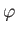). Making use of the results quoted in Section C.4, the components of the stress tensor are(1.157)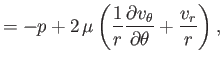(1.158)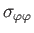(1.159)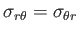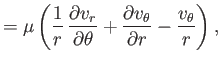(1.160)(1.161)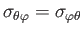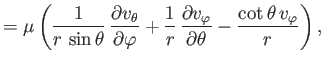(1.162)

whereas the equations of compressible fluid flow become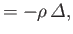(1.163)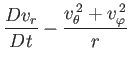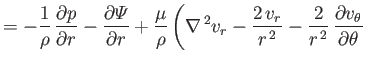(1.164)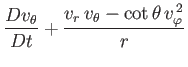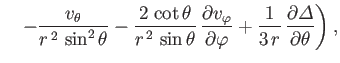(1.165)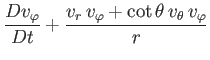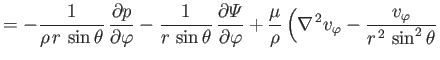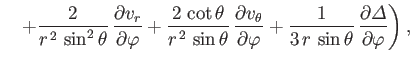(1.166)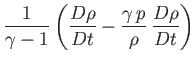(1.167)

where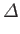(1.168)(1.169)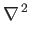(1.170)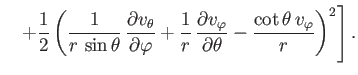(1.171)Next: Exercises Up: Mathematical Models of Fluid Previous: Fluid Equations in Cylindrical
Richard Fitzpatrick 2016-03-31